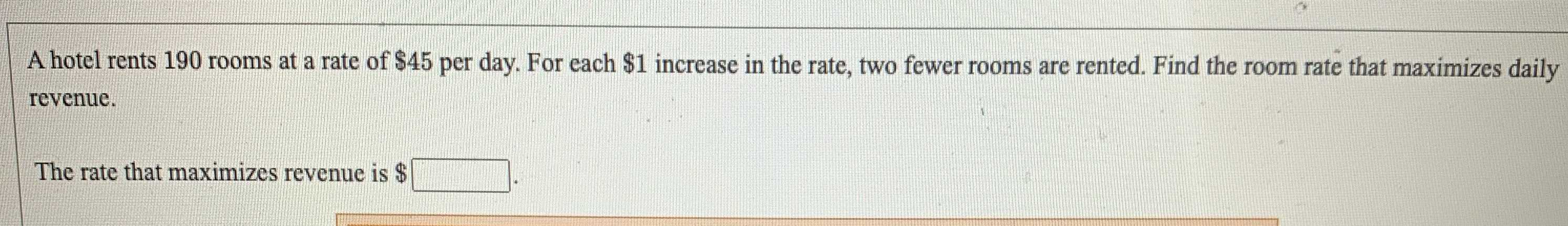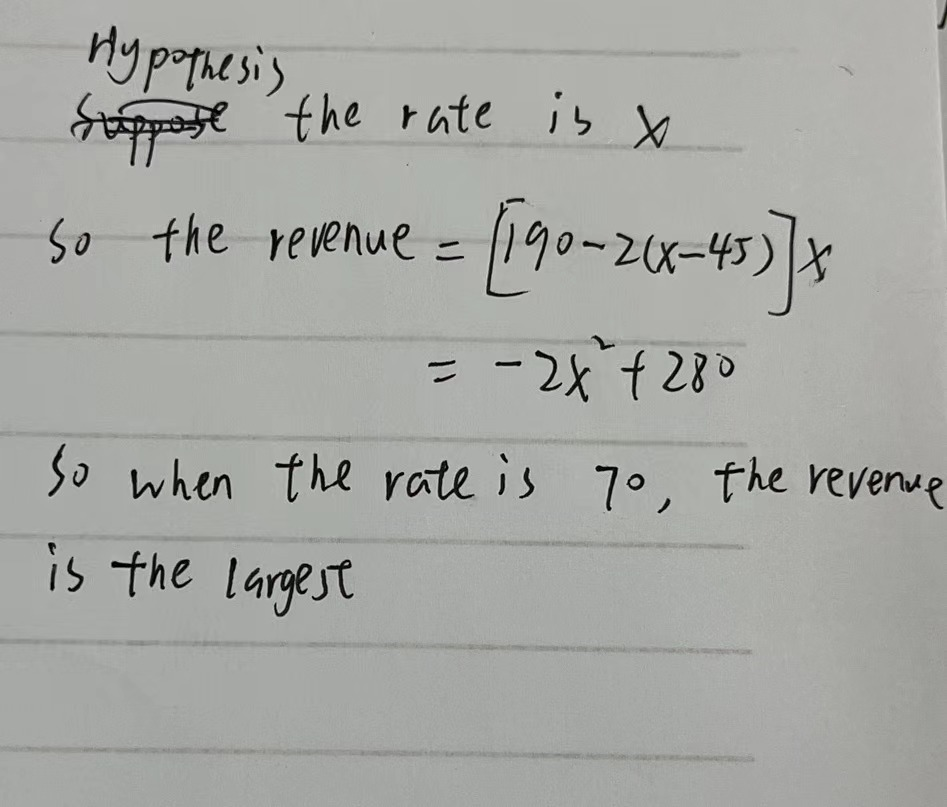### ¿Todavía tienes preguntas de matemáticas?

Pregunte a nuestros tutores expertos
Algebra
PreguntaA hotel rents $$190$$ rooms at a rate of $$\ 45$$ per day. For each $$\ 1$$ increase in the rate, two fewer rooms are rented. Find the room rate that maximizes daily revenue. The rate that maximizes revenue is $$\$$

70